Applied and Computational Mathematics
Volume 4, Issue 3, June 2015, Pages: 130-134

Empirical Analysis of the Fractal Features Analysis on London Gold Futures Market

Hong Zhang, Li Zhou, Jian Guo

School of Information, Beijing Wuzi University, Beijing, China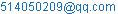(Hong Zhang)

Hong Zhang, Li Zhou, Jian Guo. Empirical Analysis of the Fractal Features Analysis on London Gold Futures Market. Applied and Computational Mathematics. Vol. 4, No. 3, 2015, pp. 130-134. doi: 10.11648/j.acm.20150403.15

Abstract: In this paper, we study the fractal characteristics of the futures market. We take the empirical study on London Gold Futures yield by Rescaled Range Analysis, analyzing the fractal characteristics of the futures market. We further determine fractal characteristics and the structure of the nonlinear time series through random disturb the original time series observation sequence. The result of R/S analysis shows that the movement of market prices of the financial markets has obvious nonperiodic circle, with Hurst index large than 0.5 and C (t) large than 0, which indicates clear fractal properties. And the result also shows that the influence of price limit on the fractal properties of London Gold Futures Market is very remarkable.

Keywords: Fractal Characteristics, Nonlinear Time Series, R/S Method

1. Introduction

The nature of social economic phenomenon is different from the natural phenomenon, in the natural phenomenon, many variables are normally distributed, and many phenomena in the social and economic phenomenon obeys the inverse power law distribution. They can be described using fractal distribution of non-normal.

The result of R/S analysis shows that the movement of market prices of the financial markets has obvious nonperiodic circle, with Hurst index large than 0.5 and C (t)large than 0, which indicates clear fractal properties. And the result also shows that the influence of price limit on the fractal properties of London Gold Futures Market is very remarkable. According to the results of the analysis of London Gold Futures Market price fluctuations cycle data, London Gold Futures Market Hurst index was 0.6392, cycle length is 208.92 days, showing obvious fractal characteristics.

There are 3 Levels titles in an article to make ideas clear:

(1)Introducing the concept of fractal;

(2)Analyzes the fractal characteristics of the futures market by R/S analysis;

(3)Further determine fractal characteristics and the structure of the nonlinear time series through random disturb the original time series.

2. Background

2.1. The Definition of Fractal

Mandelbrot in 1975, creatively put forward the concept of fractal1-3: if a collection of Hausdorff dimension is strictly greater than its topological dimension, this collection is called fractal sets1,4,5. This definition shows that the fractal is a geometric objects with flexible symmetry or expansion symmetry.the central content refers to the irregular geometry in the dynamic evolution process, in a certain scale range,the corresponding measure does not vary with scale changes.The definition will lead the study of structure deeply, but it lacks in practical operation, so it is not satisfactory.

In 1982, Fractals is given another definition:If a whole and part of the collection has a certain degree of self-similarity, this collection is called fractal.  This definition is still a lack of rigour. In fact, for what is a fractal, so far there are no precise definition.

After revision, many researchers is generally believed that the fractal has the following features:

(1) The fractal has a fine structure, in any small scale can present a more delicate details;

(2) The irregularity of the fractal both in the global and local can not be described in traditional geometrical language, it is not the locus of points that meet certain criteria,either, is not some simple equation sets;

(3) Have some form of self-similarity, fractal may be approximate self-similar or statistical self similarity;

(4) Normally, the fractal dimension of fractal sets is strictly greater than its topological dimension;

(5) In many cases, construction rules of fractal sets is very simple, perhaps by recursively generated;

(6) The fractal size cannot be measured by the usual measure.

In general, the fractal structure, there are two obvious characteristics: one is the self-similarity,which repeats zoom fractal detail (fractal) structure that can be seen is similar to itself again, and this appears random, which is scale-invariant; the second is the lack of smoothness, discontinuous everywhere, nor differential.

2.2. The Introduce of R/S Method

R/S analysis3,4,11 (Rescaled range analysis) is a kind of time series statistical method proposed by Hurst in 1965.Greene and Fielit introduced R/S analysis method into the mathematical finance,studied the behavior characteristics of common stock returns, followed by R/S analysis method is widely applied to study the fractal structure of financial time series products.the basic principle and method of R/S analysis method is as follows:

The time series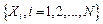is divided into int [N/n], the time length is n independent time series. Define the first m during the sample mean is: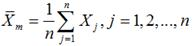(1)

Cumulative deviation: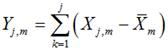(2)

Range: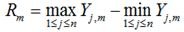(3)

Variance: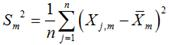(4)

For Brownian motion,statistics of R/S are as follows: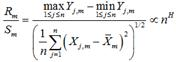(5)

3. The Empirical Analysis

3.1. The Selection of Data and Its Characteristic

Selecting London gold futures as research object. Sample interval is from 2005.01.03 to 2015.4.4. Using Logarithm yields,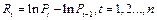The specific analysis process of the financial market returns time series by R/S analysis is: Selecting from 2 to half the length of the time interval of return series, calculating the value of the rescaled range sequence, and then calculate the V statistic value7; To determine the maximum V corresponding interval is the statistical cycle length n; using the Log sequence of rescaled range between 2 and N making ordinary least squares regression to time interval return series to estimating Hurst index.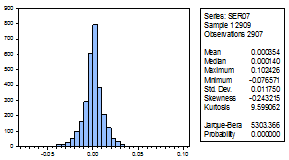Fig. 1. Normality tests.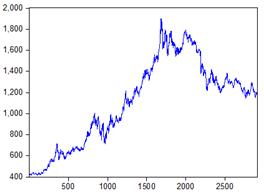Fig. 2. Daily closing price time series.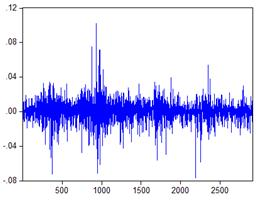Fig. 3. Daily return time series.

The results of statistics show that tail of exponential gains and losses distribution is fatter than normal distribution’s. Which mains abnormal fluctuations in the market happen sometimes, the fact that skewness is negative shows, from a long-term perspective, that fluctuation in the left side of exponential gains and losses distribution is larger than right side. So normal distribution cannot effectively characterize these phenomena.

3.2. The R/S Analysis of London Gold Futures Market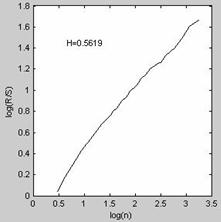Fig. 4. Daily return R/S analysis.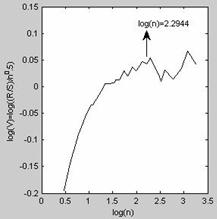Fig. 5. Daily return V statistics.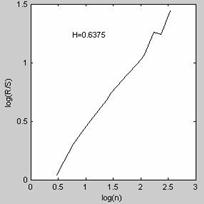Fig. 6. Weekly return R/S analysis.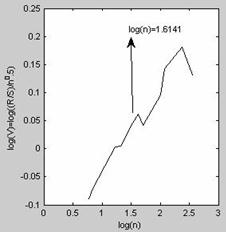Fig. 7. Weekly return V statistics.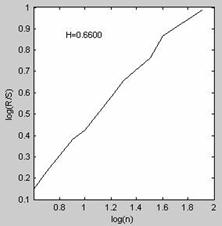Fig. 8. Monthly return R/S analysis.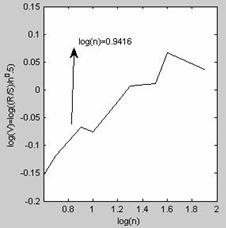Fig. 9. Monthly return V statistics.

Table 1. R/S analysis result.

 H C(t)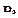Cycle Without residual treatment Daily yield 0.4508 -0.0215 1.327 196.85 Weekly yield 0.5264 0.0989 1.2514 205.50 Monthly yield 0.5489 0.1372 1.2289 200.94 residual treatment Daily yield 0.4474 -0.0266 1.3304 200.51 Weekly yield 0.5281 0.1017 1.2497 208.80

3.2.1. Data Pre-Processing Eliminating Short Term Correlation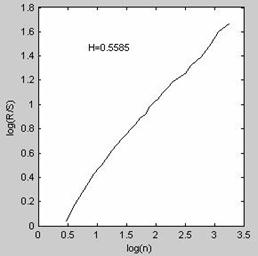Fig. 10. Daily return R/S analysis after residual treatment.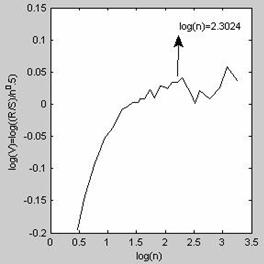Fig. 11. Weekly return R/S analysis after residual treatment.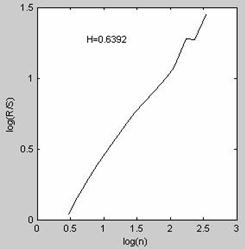Fig. 12. Monthly return R/S analysis after residual treatment.Fig. 13. Weekly return V statistics after residual treatment.

The result of daily return and weekly return by AR (1) the residual R/S analysis are as shown in figure 10---figure 13 respectively.Compare figure 4 and 10, you can see that compared with the original daily yield sequence of Hurst index, after dealing with the AR (1), Hurst index decreases. Suggesting that there exist short-term correlation with daily yield sequence.and AR (1) treatment can eliminate or reduce the correlation within short-term yield sequence.Compare figure 6 and figure 12,compared with Hurst index obtained through the original weekly returns time series, after dealing with the AR (1), Hurst index basically unchanged.Showing short-term correlation of  weekly returns time series is more lesser,and it is different from the daily return series.

3.2.2. Results Analysis

Based on the London Gold futures prices time series R/S analysis, can get the following conclusion:

1)there are sustainability with futures price volatility. By the above results, It shows that London Gold futures price time series is not a random walk, but with persistence,However, such persistence is not permanent,and will disappear after a period of time. With the sample data time frequency increased, Hurst index8,9,12 decreased,suggesting that daily returns data has more noise,comparing with weekly data and monthly data, daily return figures more volatile.so it's more unstable.

2) there exist association with futures price volatility.according to the analysis results,c (t) of daily returns, weeks yields and mouthly yields data are greater than 0,so that futures returns is positive correlation, the futures price fluctuation is not only affected by the future information, and the impact of current and past information to the futures price is very obvious.

3) there exist periodicity with futures price volatility.According to fig.4 ~ fig.9 can be seen,The reason of there is a certain gap between the daily yield and weekly yield,monthly yield is the fact that daily returns data has more noise.

4) Daily returns than weekly yields with higher short-term correlation.compared with Hurst index obtained through the original weekly returns time series, after dealing with the AR (1), Hurst index basically unchanged.Showing short-term correlation of  weekly returns time series is more lesser,and it is different from the daily return series.

4. Test of R/S Analysis 5,6,10

4.1. The Upset Test of R/S Analysis

As you can be seen from figure 3-43, the Hurst index fell to 0.6029 after upset,and the slope of the regression curve of disturbed sequence with reduced to a certain extent,showing that it has destroyed the structure of the original time series, but has not yet been transformed into a truly independent sequence.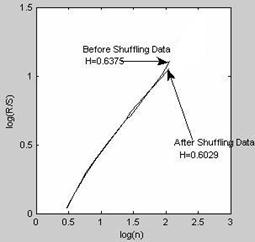Fig. 14. Daily return time series.

4.2. To Test the Reliability of R/S Analysis

Table 2. The reliability of R/S analysis test result.

 Data Weekly yield H 0.5281 E(H) 0.4013 -0.0737 Significant 3.2789

5. Summary

First of all, the paper introduces the concept of fractal. studying price fluctuation in the futures market using R/S analysis method, the results show that Hurst index of the price volatility were significantly greater than 0.5, the correlation coefficient C (t) are greater than zero, and has obvious non-periodic cycle, this suggests that the price fluctuation in the financial market have obvious relevance and long-range correlation, has obvious fractal characteristics.

Acknowledgements

This project (Empirical research on Stock index investment risk model, No.68) is funded by the "2014-2015 school year, Beijing Wuzi University, College students' scientific research and entrepreneurial action plan project". And by Beijing Wuzi University Yunhe scholars program(00610303/007). And by Beijing Wuzi UniversityManagement science and engineering Professional group of construction projects.(No.PXM2015_014214_000039)

References

1. Terence C. Mills. Statistical analysis of high frequency data from the Athens stock exchange [J].Physica A, 2001, 293: 566-572.
2. Marc-Etienne Brachet, Erik Taflin, Jean Marcel Tcheou. Scaling transformation and probability distributions for financial time series [J]. Chaos, Solitons and Fractals, 2000, 11: 2343-2348.
3. Verslin M. C., Belward J. A., Fractal dimensions for rainfall time series [J],Mathematics and computers in Simulation, 1999(48): 437~446
4. Ge Shirong, Chen Guoan, Fractal prediction models of sliding wear during the running in proecess[J], Wear, 1999(231): 249~255.
5. Fisher, A., Calvert L., Mandelbrot, B. B., Multifractality of deutschmark/US dollar exchange rates[R], Working paper, Yale university, 1997
6. Mandelbrot B. B., Fisher A., Calvert L., A multifractal model of asset returns[R], Working paper, Yale university, 1997.
7. Chung Yuan, Statistical properties of volatility in fractal dimension andprobability distribution among six stock markets---USA . Japan, Taiwan, South,Korea, Singapore, and HongKong[R], Working paper, New York, SSRN, id425300, 2004
8. Cornelis A. Los, Measuring the degree of financial market efficiency: An essay[R], Working paper, New York, SSRN, id614544, 2004
9. Yan Olszewski, Building a better fund of hedge funds: a fractal and α-stable distribution approach[R] , Working paper, New York, SSRN, id776064, 2004.
10. Lawrence A. Cunningham, From random walks to chaotic crashes: the linear genealogy of the efficient capital market hypothesis[R], Working paper, New York, SSRN, id 244670, 2004.
11. Cornelis A. Los, Rossitsa Yalamova, Multifractal spectral analysis of the 1987 stock[R], Working paper, New York, SSRN, id588823, 2004.

 Contents 1. 2. 2.1. 2.2. 3. 3.1. 3.2. 4. 4.1. 4.2. 5.
Article ToolsAbstractPDF(3395K)